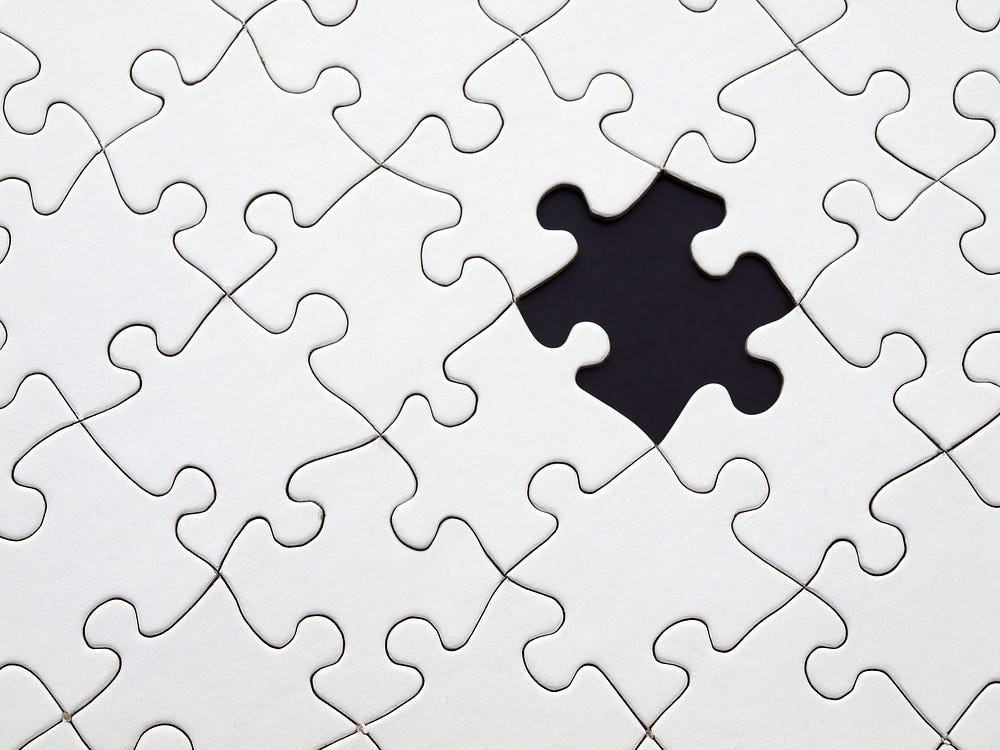# New to CNN? Learn your basics here with the MNIST Digit Recognizer dataset!

Source: Deep Learning on Medium# Introduction

This is a 5 layers Sequential Convolutional Neural Network for digits recognition trained on MNIST dataset. I choosed to build it with keras API (Tensorflow backend) which is very intuitive. Firstly, I will prepare the data (handwritten digits images) then i will focus on the CNN modeling and evaluation.

I achieved 99.671% of accuracy with this CNN trained in 2h30 on a single CPU (i5 2500k). For those who have a >= 3.0 GPU capabilites (from GTX 650 — to recent GPUs), you can use tensorflow-gpu with keras. Computation will be much much faster !!!

For computational reasons, i set the number of steps (epochs) to 2, if you want to achieve 99+% of accuracy set it to 30.

I’ll jump straight to the code, that’s where you can experiment and learn what different combinations of parameters do for you and take a look at how the data responds to it!

`import pandas as pdimport numpy as npimport matplotlib.pyplot as pltimport matplotlib.image as mpimgimport seaborn as sns%matplotlib inlinenp.random.seed(2)from sklearn.model_selection import train_test_splitfrom sklearn.metrics import confusion_matriximport itertoolsfrom keras.utils.np_utils import to_categorical # convert to one-hot-encodingfrom keras.models import Sequentialfrom keras.layers import Dense, Dropout, Flatten, Conv2D, MaxPool2Dfrom keras.optimizers import RMSpropfrom keras.preprocessing.image import ImageDataGeneratorfrom keras.callbacks import ReduceLROnPlateausns.set(style='white', context='notebook', palette='deep')`

# Data preparation

`# Load the datatrain = pd.read_csv("../input/train.csv")test = pd.read_csv("../input/test.csv")Y_train = train["label"]# Drop 'label' columnX_train = train.drop(labels = ["label"],axis = 1) # free some spacedel train g = sns.countplot(Y_train)Y_train.value_counts()`